NEET  >  Previous Year Questions: Motion in a Straight Line

# Previous Year Questions: Motion in a Straight Line

Test Description

## 28 Questions MCQ Test | Previous Year Questions: Motion in a Straight Line

Previous Year Questions: Motion in a Straight Line for NEET 2023 is part of NEET preparation. The Previous Year Questions: Motion in a Straight Line questions and answers have been prepared according to the NEET exam syllabus.The Previous Year Questions: Motion in a Straight Line MCQs are made for NEET 2023 Exam. Find important definitions, questions, notes, meanings, examples, exercises, MCQs and online tests for Previous Year Questions: Motion in a Straight Line below.
Solutions of Previous Year Questions: Motion in a Straight Line questions in English are available as part of our course for NEET & Previous Year Questions: Motion in a Straight Line solutions in Hindi for NEET course. Download more important topics, notes, lectures and mock test series for NEET Exam by signing up for free. Attempt Previous Year Questions: Motion in a Straight Line | 28 questions in 28 minutes | Mock test for NEET preparation | Free important questions MCQ to study for NEET Exam | Download free PDF with solutions
 1 Crore+ students have signed up on EduRev. Have you?
Previous Year Questions: Motion in a Straight Line - Question 1

### If a body losses half of its velocity on penetrating 3 cm in a wooden block, then how much will it penetrate more before coming to rest?  [AIEEE 2002]

Detailed Solution for Previous Year Questions: Motion in a Straight Line - Question 1

Let the initial velocity of the body at point A is v, AB is 3 cm.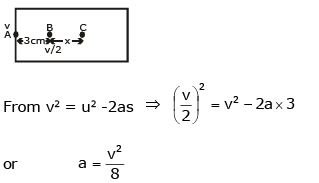On penetrating 3 cm in a wooden block, let the body moves x distance from B to C.
For B to C: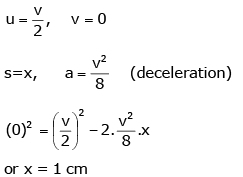Note: Here, it is assumed that retardation is uniform.

Previous Year Questions: Motion in a Straight Line - Question 2

### From a building, two balls A and B are thrown such that A is thrown upwards and B downwards (both vertically with the same velocity). If vA and vB are their respective velocities on reaching the ground, then:    [AIEEE 2002]

Detailed Solution for Previous Year Questions: Motion in a Straight Line - Question 2

From the conservation of energy, potential energy at height h = kinetic energy at the ground.
Therefore, at height h, PE of ball A, PE = mAgh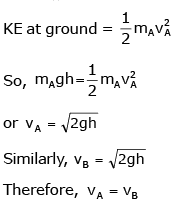Previous Year Questions: Motion in a Straight Line - Question 3

### A ball whose kinetic energy is E is projected at an angle of 45° to the horizontal. The kinetic energy of the ball at the highest point of its flight will be:          [AIEEE 2002]

Detailed Solution for Previous Year Questions: Motion in a Straight Line - Question 3

At the highest point of its flight, the vertical component of velocity is zero and the only horizontal component is left which is ux = u cosθ
Given θ = 450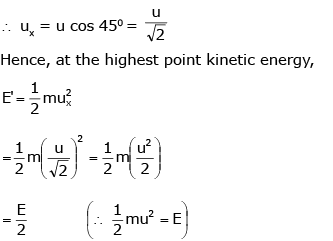Previous Year Questions: Motion in a Straight Line - Question 4

Speeds of two identical cars u and 4u at a specific instant. The ratio of the respective distances at which the two cars are stopped from that instant by the same braking force:

[AIEEE 2002]

Detailed Solution for Previous Year Questions: Motion in a Straight Line - Question 4

In this question, the cars are identical means the coefficient of friction between the tyre and the ground is the same for both the cars, as a result, retardation is the same for both the cars equal to mg.
Let first car travel distance s1, before stopping while second car travel distance s2, then from v2 = u2 - 2as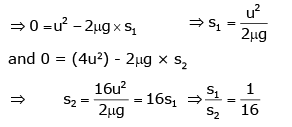Previous Year Questions: Motion in a Straight Line - Question 5

The coordinates of a moving particle at any time t are given by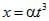and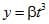. The speed of the particle at time t is given by:

[AIEEE 2003]

Detailed Solution for Previous Year Questions: Motion in a Straight Line - Question 5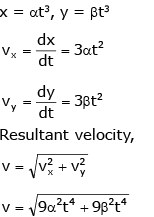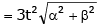Previous Year Questions: Motion in a Straight Line - Question 6

A boy playing on the roof of a 10 m high building throws a ball with a speed of 10 m/s at an angle of 30° with the horizontal. How far from the throwing point will the ball be at the height of 10 m from the ground? [g = 10 m/s2, sin 30° = 1/2, cos 30° = √3/2]

[AIEEE 2003]

Detailed Solution for Previous Year Questions: Motion in a Straight Line - Question 6

We have to simply calculate the range of the projectile.
► R = u2sin2θ/g
► R = (10)2 * sin (2 * 30º) / 10
► R = 10 * (√3/2) = 8.66 m

Previous Year Questions: Motion in a Straight Line - Question 7

A car moving with a speed of 50 km/h, can be stopped by brakes after at least 6 m. If the same car is moving at a speed of 100 km/h, the minimum stopping distance is:

[AIEEE 2003]

Detailed Solution for Previous Year Questions: Motion in a Straight Line - Question 7

We know that retardation of a car with an initial speed of 50kmph stops after 6 m.
We know that v2 - u2 = 2as  (third equation of motion)
► 0 - 502 = 2 * a * 6
► a = - 502 / 12
Hence when the initial speed is 100 kmph
We get,
► -1002 = 2 * a * s
► 2 * s = 12 * (1002 / 502)
Thus we get s = 24 m.

Previous Year Questions: Motion in a Straight Line - Question 8

A ball is thrown from a point with a speed v0 at an angle of projection θ. From the same point and at the same instant, a person starts running with a constant speed v0 / 2 to catch the ball. Will the person be able to catch the ball? If yes, what should be the angle of projection?

[AIEEE 2004]

Detailed Solution for Previous Year Questions: Motion in a Straight Line - Question 8

For a person to catch the ball they should travel the same distance along the horizontal axis in time T.
Distance covered by person, R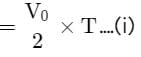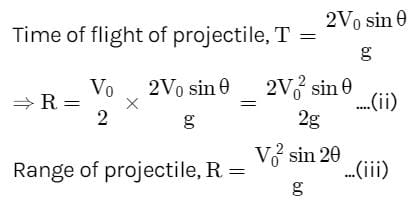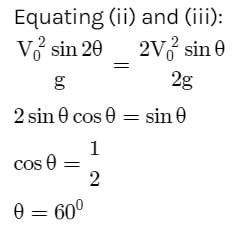Previous Year Questions: Motion in a Straight Line - Question 9

A particle is acted upon by a force of constant magnitude which is always perpendicular to the velocity of the particle. The motion of the particle takes place in a plane, it follows that:

[AIEEE 2004]

Detailed Solution for Previous Year Questions: Motion in a Straight Line - Question 9

When a force of constant magnitude acts on the velocity of a particle perpendicularly, then there is no change in the kinetic energy of the particle. Hence, kinetic energy remains constant.

Previous Year Questions: Motion in a Straight Line - Question 10

An automobile travelling at a speed of 60 km/h, can apply the brake to stop within a distance of 20 m. If the car is going twice as fast, i.e. 120 km/h, the stopping distance will be:

[AIEEE 2004]

Detailed Solution for Previous Year Questions: Motion in a Straight Line - Question 10
• We know that with retardation of a car with an initial speed of 60 kmph stops after 20m.
• We know that v2 = 2as  (third equation of motion). Thus we get that for some constant retardation, stopping distance is proportional to the square of the initial speed.
• Hence when the initial speed is 120kmph we get,
s = 20 x (120/60)2
s = 80 m
Previous Year Questions: Motion in a Straight Line - Question 11

A ball is released from the top of a tower of height h meter. It takes T seconds to reach the ground. What is the position of the ball in T/3 seconds?

[AIEEE 2004]

Detailed Solution for Previous Year Questions: Motion in a Straight Line - Question 11

Second law of motion gives: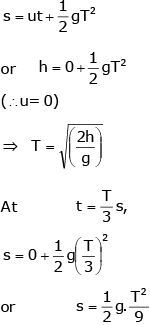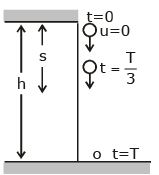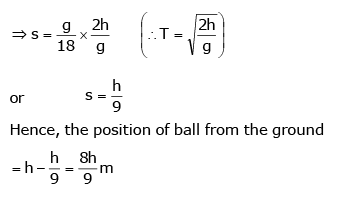Previous Year Questions: Motion in a Straight Line - Question 12

A parachutist after bailing outfalls 50 m without friction. When the parachute opens, it decelerates at 2 ms-2. He reaches the ground with a speed of 3 ms-1. At what height, did he bail out?

[AIEEE 2005]

Detailed Solution for Previous Year Questions: Motion in a Straight Line - Question 12

Parachute bails out at height H from ground. Velocity at A: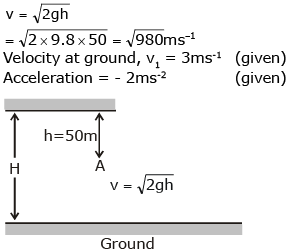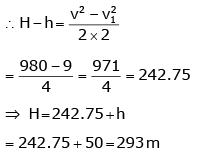Previous Year Questions: Motion in a Straight Line - Question 13

A particle is moving eastwards with a velocity of 5 ms-1. In 10 s the velocity changes to 5 ms-1 northwards. The average acceleration in this time is:

[AIEEE 2005]

Detailed Solution for Previous Year Questions: Motion in a Straight Line - Question 13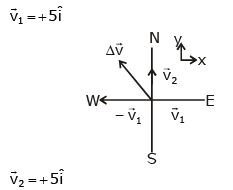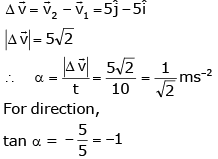The average acceleration is 1/√2 ms-2 towards the northwest.

Previous Year Questions: Motion in a Straight Line - Question 14

A car, starting from rest, accelerates at the rate f through a distance s, then continues at a constant speed for time t and then decelerates at the rate f/2 to come to rest. If the total distance travelled is 15 s, then:

[AIEEE 2005]

Detailed Solution for Previous Year Questions: Motion in a Straight Line - Question 14

The velocity-time graph for the given situation can be drawn as below. Magnitudes of the slope of OA = f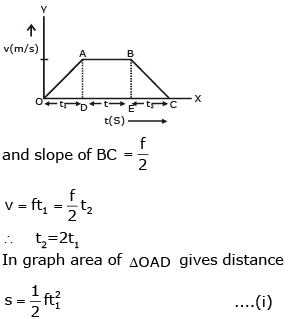Area of rectangle ABED gives distance travelled in time t.
► s2 = (ft1) * t
Distance travelled in time t2,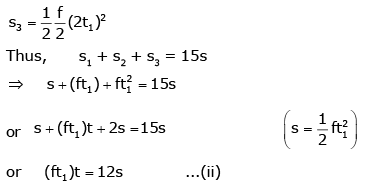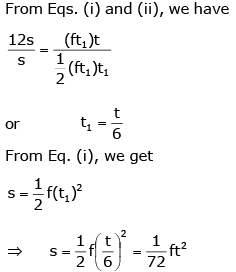Previous Year Questions: Motion in a Straight Line - Question 15

The relation between time t and distance x is t = ax2 + bx, where a and b are constants. The acceleration is:

[AIEEE 2005]

Detailed Solution for Previous Year Questions: Motion in a Straight Line - Question 15

Given, t = ax+ bx
Differentiating w.r.t  t, we get: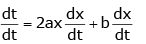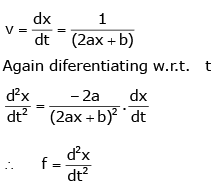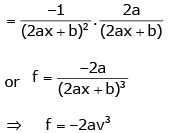Previous Year Questions: Motion in a Straight Line - Question 16

A projectile can have the same range R for two angles of projection. If t1 and tbe the times of flights in the two cases, then the product of the two times of flights is proportional to:

[AIEEE 2005]

Detailed Solution for Previous Year Questions: Motion in a Straight Line - Question 16

A projectile can have the same range if the angle of projection are complementary ie, θ and (90- θ). Thus, in both cases: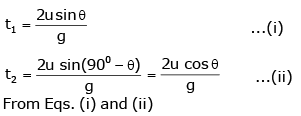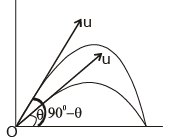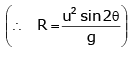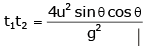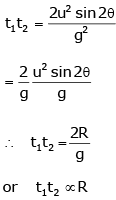Previous Year Questions: Motion in a Straight Line - Question 17

A particle located at x = 0 at time t = 0, starts moving along the positive x-direction with a velocity v that varies as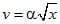. The displacement of the particle varies with time as:

[AIEEE 2006]

Detailed Solution for Previous Year Questions: Motion in a Straight Line - Question 17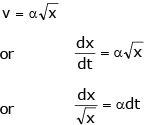On performing integration: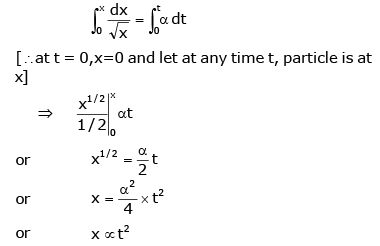Previous Year Questions: Motion in a Straight Line - Question 18

A particle is projected at 60° to the horizontal with a kinetic energy K. The kinetic energy at the highest point is:

[AIEEE 2007]

Detailed Solution for Previous Year Questions: Motion in a Straight Line - Question 18

Kinetic energy at highest point,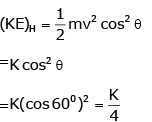Previous Year Questions: Motion in a Straight Line - Question 19

The velocity of a particle is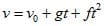. If its position is x = 0 at t = 0, then its displacement after unit time (t = 1) is:

[AIEEE 2007]

Detailed Solution for Previous Year Questions: Motion in a Straight Line - Question 19

v = v​+ gt + ft2
0x​ dx = ∫01​(v0​ + gt + ft2) dt
► x = v0 ​+ g/2 ​+ f​/3

Previous Year Questions: Motion in a Straight Line - Question 20

A body is at rest at x = 0. At t = 0, it starts moving in the positive x-direction with a constant acceleration. At the same instant, another body passes through x = 0 moving in the positive x-direction with a constant speed. The position of the first body is given by x1 (t) after time t and that of the second body by x2(t) after the same time interval. Which of the following graphs correctly describes (x1 – x2) as a function of time?

[AIEEE 2008]

Detailed Solution for Previous Year Questions: Motion in a Straight Line - Question 20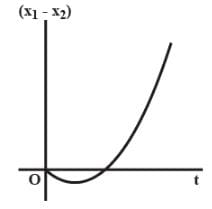► x1​(t) = 1​/2 at2
► x2​(t) = vt
► (x1 - x2) = 1/2​ at− vt
Now Plot (x1​−x2) vs t

Previous Year Questions: Motion in a Straight Line - Question 21

Consider a rubber ball freely falling from a height h = 4.9 m onto a horizontal elastic plate. Assume that the duration of the collision is negligible and the collision with the plate is totally elastic. Then the velocity as a function of time the height as a function of time will be:
[AIEEE 2009]

Detailed Solution for Previous Year Questions: Motion in a Straight Line - Question 21
• We know, v = u + gt
As the ball is dropped, v = gt.
When coming down, v increases then makes collision, the value of v becomes +ve, then value of v decreases, becomes zero and then increases.
• The change from +v to –v is almost instantaneous. Using -ve signs when coming down, so option c is correct.
Further h = (1/2) gt2  is a parabola. Therefore (c) is correct.
Previous Year Questions: Motion in a Straight Line - Question 22

A particle has initial velocity (2i + 3j) and acceleration (0.3i + 0.2j). The magnitude of velocity after 10 s will be:

Detailed Solution for Previous Year Questions: Motion in a Straight Line - Question 22

From the equation of motion, we knows,
v = u + at  ...(1)
► u = (2i + 3j)
► a = (0.3i + 0.2j)
► t = 10 s
On putting these values in first equation we get:
v = (2i + 3j) + (0.3i + 0.2j) * 10
v = 5i + 5j
|v| = 5√2

Previous Year Questions: Motion in a Straight Line - Question 23

A small particle of mass m is projected at an angle θ with the x-axis with an initial velocity v0 in the x-y plane as shown in the figure. At a time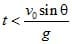, the angular momentum of the particle is:

[AIEEE 2010]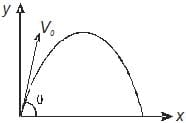Detailed Solution for Previous Year Questions: Motion in a Straight Line - Question 23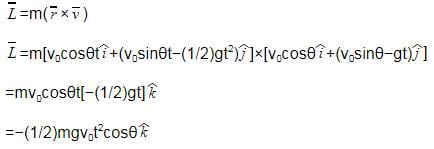Previous Year Questions: Motion in a Straight Line - Question 24

A particle is moving with velocity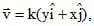where k is a constant. The general equation for its path is:

[AIEEE 2010]

Detailed Solution for Previous Year Questions: Motion in a Straight Line - Question 24

As, v = k(yi + xi)
vx = Ky → dx/dt = Ky
v= Kx → dy/dt = Kx
Dividing both equations,
► dy/dx = dy/dt divided by dx/dt = Kx / Ky = x/y
► ydy = xdx
Integrating the equation,
► y2/ 2 = x2/2 + c
► y= x+ constant

Previous Year Questions: Motion in a Straight Line - Question 25

A water fountain on the ground sprinkles water all around it. If the speed of water coming out of the fountain is v, the total area around the fountain that gets wet is:

[AIEEE 2011]

Detailed Solution for Previous Year Questions: Motion in a Straight Line - Question 25

The maximum range of water coming out of the fountain,
Rm = v2/g
Total area around fountain,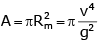Previous Year Questions: Motion in a Straight Line - Question 26

An object, moving with a speed of 6.25 m/s, is decelerated at a rate given by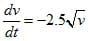where v is the instantaneous speed. The time taken by the object, to come to rest, would be:

[AIEEE 2011]

Detailed Solution for Previous Year Questions: Motion in a Straight Line - Question 26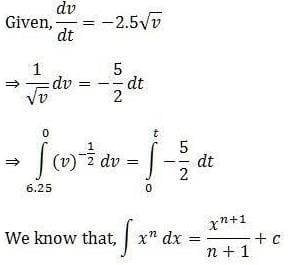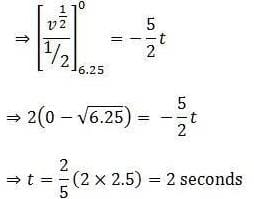Previous Year Questions: Motion in a Straight Line - Question 27

A boy can throw a stone up to a maximum height of 10 m. The maximum horizontal distance that the boy can throw the same stone up to will be:

[AIEEE 2012]

Detailed Solution for Previous Year Questions: Motion in a Straight Line - Question 27

Maximum speed with which the boy can throw stone is: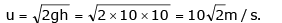Range is maximum when the projectile is thrown at an angle of 450 thus,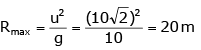Previous Year Questions: Motion in a Straight Line - Question 28

A particle of mass m is at rest at the origin at time t = 0. It is subjected to a force F(t) = F0e-bt in the x-direction. Its speed v(t) is depicted by which of the following curves?

[AIEEE 2012]

Detailed Solution for Previous Year Questions: Motion in a Straight Line - Question 28

As the force is exponentially decreasing, so its acceleration, i.e., rate of increase of velocity will decrease with time. Thus, the graph of velocity will be an increasing curve with decreasing slope with time.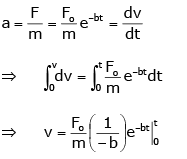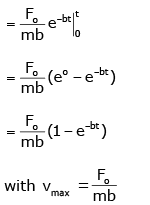Use Code STAYHOME200 and get INR 200 additional OFF Use Coupon Code
Information about Previous Year Questions: Motion in a Straight Line Page
In this test you can find the Exam questions for Previous Year Questions: Motion in a Straight Line solved & explained in the simplest way possible. Besides giving Questions and answers for Previous Year Questions: Motion in a Straight Line, EduRev gives you an ample number of Online tests for practice

### How to Prepare for NEET

Read our guide to prepare for NEET which is created by Toppers & the best Teachers